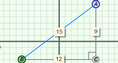# Distance Formula

### DESCRIPTION

Explore the distance formula as an application of the Pythagorean theorem. Learn to see any two points as the endpoints of the hypotenuse of a right triangle. Drag those points and examine changes to the triangle and the distance calculation.

Full Lesson Info